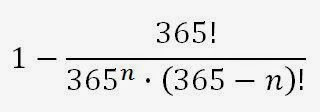## Saturday, 19 April 2014

### The Surprising Probability of Shared Birthdays

Have you ever wondered why, in a group of maybe a few dozen people (say a class of students or the staff in an office), it is fairly common for there to be a shared birthday? There are 365 days in a year but only 20 to 30 people in a typical elementary school classroom. Commonsense says shared birthdays in a small group ought to be rare, right?

When it comes to understanding probability and randomness, our commonsense often leads us astray. Our brains are better suited at comprehending patterns, structure, and order, so much so that when faced with chaos and randomness we tend to search for patterns and attempt to impose order. Our belief of what a random sample should look like is often not very random at all. So when we have a group of 20 random people, we'd like to believe that their birthdays should be evenly distributed throughout the year.

Let's analyze the shared birthday problem and find out how probable shared birthdays really are. First off, what are the chances that two randomly selected people have the same birthday? This is a rather straightforward problem, assuming that birthdays are evenly distributed among the 365 calendar days (let's neglect those leap-year birthdays). The probability is 1/365 (about 0.27%). Nothing counter-intuitive about that; our gut feeling should be that it's unusual for two random people to have the same birthday.

Where our intuition starts to lead us astray is when we start adding more random people to the sample. Let's go up to three people now (A, B, and C). There is a 1/365 chance that B has the same birthday as A. Equivalently, there's a 364/365 chance that B doesn't share a birthday with A. Having taken up two days of the year with A and B means that C has a 363/365 chance of not sharing a birthday with either A or B. The probability of there being no shared birthday in the group is therefore [364/365] * [363/365] (about 99.18%). To find the probability of there being a shared birthday, just subtract the probability of there being no shared birthday from 100%. In other words, there's about a 0.82% chance that there is a shared birthday among three randomly selected people. The probability is small, but keep in mind all we did was add a third person and we nearly tripled the probability of a shared birthday in the group. If we add a fourth person, we get a probability of 1 - {[364/365] * [363/365] * [362/365]}, which is about 1.64%. That's about double the chance of a shared birthday in the group of three.

We can calculate the probability of there being a shared birthday in any size of random sample using the following complicated-looking equation:Probability of a shared birthday in a group of n randomly selected people.
where n is the number of randomly selected people in the group and the ! indicates the factorial function. The probability of a shared birthday rapidly approaches 100% because that 365^n factor in the denominator makes that whole fraction really small really fast as n increases. You can see for yourself if you plot the equation at different value of n like I have below:Looking at it in a slightly different way, we can show what's the minimum size of group you need to have for a given chance of there being a shared birthday:

The results may be surprising. You might find them hard to believe. Intuitively, we know that a group of 366 or more people must have 100% probability of a shared birthday, and a group of 1 person has a 0% probability of a shared birthday. But beyond that, most folks' intuition is way out to lunch. You probably didn't guess that with just 23 people, it is more likely than not (50.73% chance) that there is a shared birthday in the group, or that in a group of 70 people there's only about a 1 in 1000 chance of there not being a shared birthday. But now that you've seen the analysis, hopefully it's no longer surprising that there were shared birthdays in your classes or workplace.